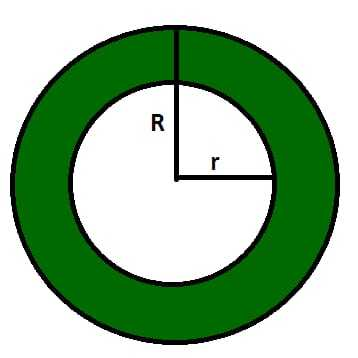# Mensuration 3D- Hollow sphere

• Last Updated : 12 Jun, 2020

In this article we shall calculate the volume, Curved surface area (CSA) and Total surface area of a hollow sphere or a spherical shell. Below shown is a diagram of a hollow sphere.As we can see in the figure, the outer radius of hollow sphere is ‘R’ and the inner radius is ‘r’.

Volume of hollow sphere :
Volume of a 3D figure is defined as the capacity of the figure or the amount of the content it can hold. The volume of hollow sphere is equal to the volume of inner sphere subtracted from the volume of the outer sphere. It can be calculated as –

We know that volume of sphere,

`= 4/3 πR3 `

Thus volume of hollow sphere= volume of outer sphere- volume of inner sphere

```= 4/3 πR3-4/3 πr3
= 4/3 π(R3-r3) ```

Curved surface area (CSA) :
The Curved surface area of hollow sphere is the area of the paper that can completely cover the surface of the hollow sphere. It is equal to the CSA of inner sphere subtracted from the CSA of outer sphere.

```CSA of hollow sphere,
= CSA of outer sphere - CSA of inner sphere
= 4 πR2 - 4 πr2
= 4 π(R2-r2) ```

Total surface area of hollow sphere :
The total surface area of a hollow sphere is equal to the CSA of hollow sphere as a hollow sphere has only one surface that constitutes it.
Thus CSA=TSA for a hollow sphere

Example-1:
Calculate the volume and TSA of hollow sphere with outer and inner radii 5cm and 3cm respectively.

Explanation :

```Given outer radius = R = 5cm
Inner radius = r = 3cm

Volume = 4/3 π(R3-r3)
= 4/3*3.14*(125-27)
= 410.29 cm3

TSA = 4 π(R2-r2) = 4*3.14*(25-9)
= 200.96 cm2 ```

Example-2:
Water is being poured into hollow sphere of outer radius 10 cm and inner radius 5 cm at the rate of 10 cm3/s. Calculate the time required to fill the hollow sphere.

Explanation :

```Outer radius = R = 10 cm
Inner radius = r = 5 cm

Volume of hollow sphere = 4/3*3.14*(1000-125)
= 3500 cm3

Rate at which water is being poured = 10 cm3/s
Time required = Volume of sphere/Rate of pouring water
= 3500/10 = 350 s ```
My Personal Notes arrow_drop_up
Recommended Articles
Page :